# Skip Counting by 5

Skip Counting by 5• To skip count in fives, keep adding 5 to get to the next number.
• We do not count the numbers in between.
• We can teach skip counting in fives using a number grid to see a pattern.
• When skip counting by 5 from zero, all of the numbers end in 5 or 0.
• The numbers are all in the same columns of the number grid.

To skip count by five, keep adding five to get to the next number.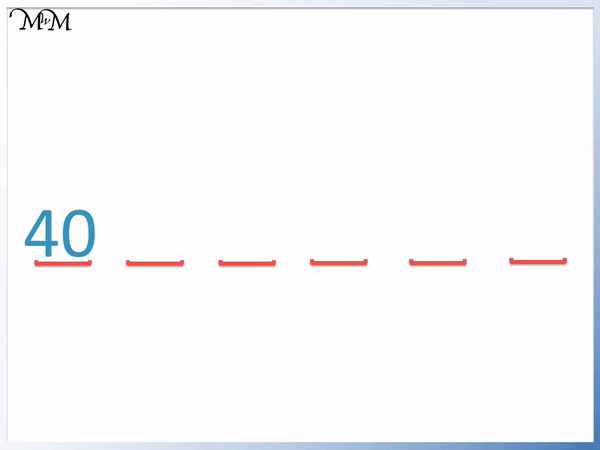• To skip count in fives, add five to get to the next number.
• We will count up in fives from 40.
• The numbers will alternative between ending in a 0 and ending in a 5.
• Adding 5 to the numbers ending in 5 in the units column takes us up to the next ten.
• There are a pair of numbers ending in 0 and then 5 for each ten.Supporting Lessons

#### Skip Counting by 5: Accompanying Lesson Worksheet# How to Skip Count by 5

## Skip Counting Up in Fives

To skip count by 5, add five to get to the next number. Keep adding 5 to each number to get to the next and do not count the numbers in between.

We start at zero and continue to add five to get to the next numbers.

When skip counting in fives from zero, the first few numbers are simply the numbers in the five times table.

We have: 5, 10, 15, 20, 25, 30, 35, 40, 45, 50, 55, 60, 65, 70, 75, 80, 85, 90, 95 and 100.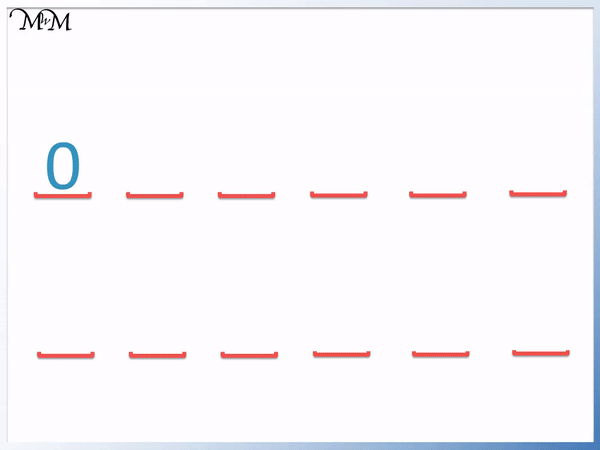To teach skip counting to children it is useful to use a number grid to 100.

Below is a number grid showing skip counting in fives.We can see that there is a pattern when skip counting in fives that can make it easy to learn.

Firstly, the numbers all fall into two columns on the number grid, which can be used to help children to find the next number in the pattern. It can help to say the numbers as you read them and even point at them on the number grid.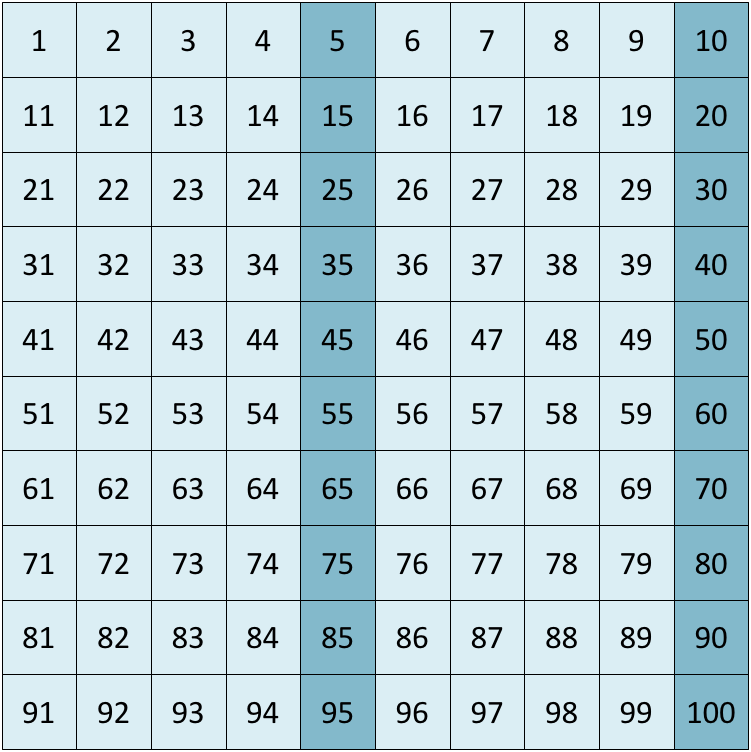Secondly, the numbers all end in 5 and then 0.

When counting up in fives from zero, the numbers alternate between ending in 5 and ending in 0.

To learn the pattern easily, we can see that each group of ten has a number ending in 0 and then 5. After each pair of 0 and 5, we reach the next ten.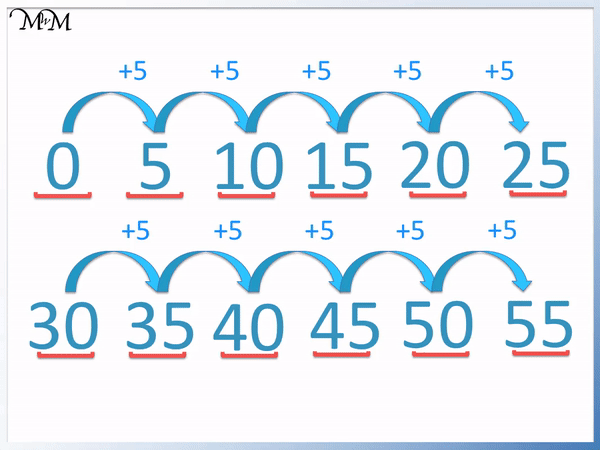We have 0 and 5, 10 and 15, 20 and 25, 30 and 35, 40 and 45, 50 and 55, 60 and 65, 70 and 75, 80 and 85, 90 and 95 and then finally, 100.

The digit in the tens column increases by 1 after adding five to each number that ends in a 5.

We can use this pattern to skip count in fives from any number.

For example, we will start at 40 and skip count forward by 5.We can use the same pattern before to help us. The numbers end in 0 and then 5. After each number ending in 5, the tens digit increases by 1.

We have 40 and 45, 50 and 55 and then 60 and 65.

There is a pair of numbers in each ten that end in a 0 and then a 5.

In this example we will count up in fives from 15.

15 ends in a 5 and so the next number will be in the next ten up and it will end in a 0 in the units column.We have: 20 and 25, 30 and 35 followed by 40.

To practise skip counting by 5, we can use skip counting caterpillar worksheets.

We will add 5 to get to the next number along and write the numbers in each segment of the caterpillar.

We fill in the blank spaces between 5 and 85.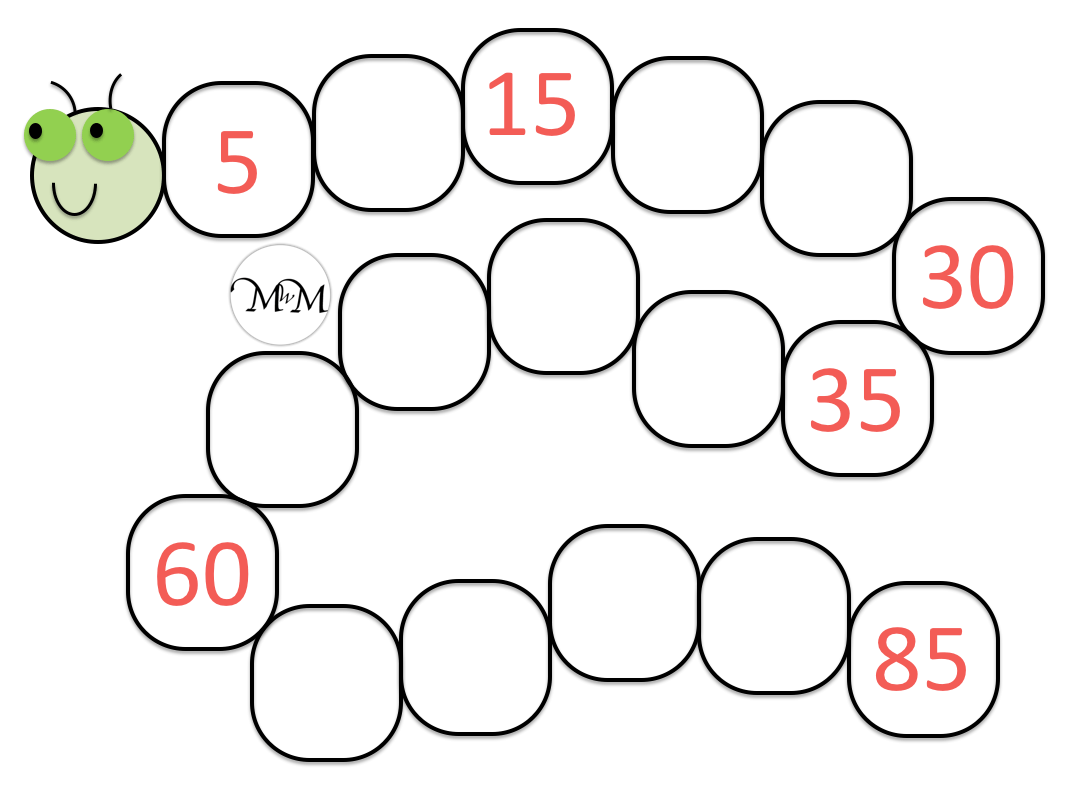Here is the caterpillar worksheet being completed.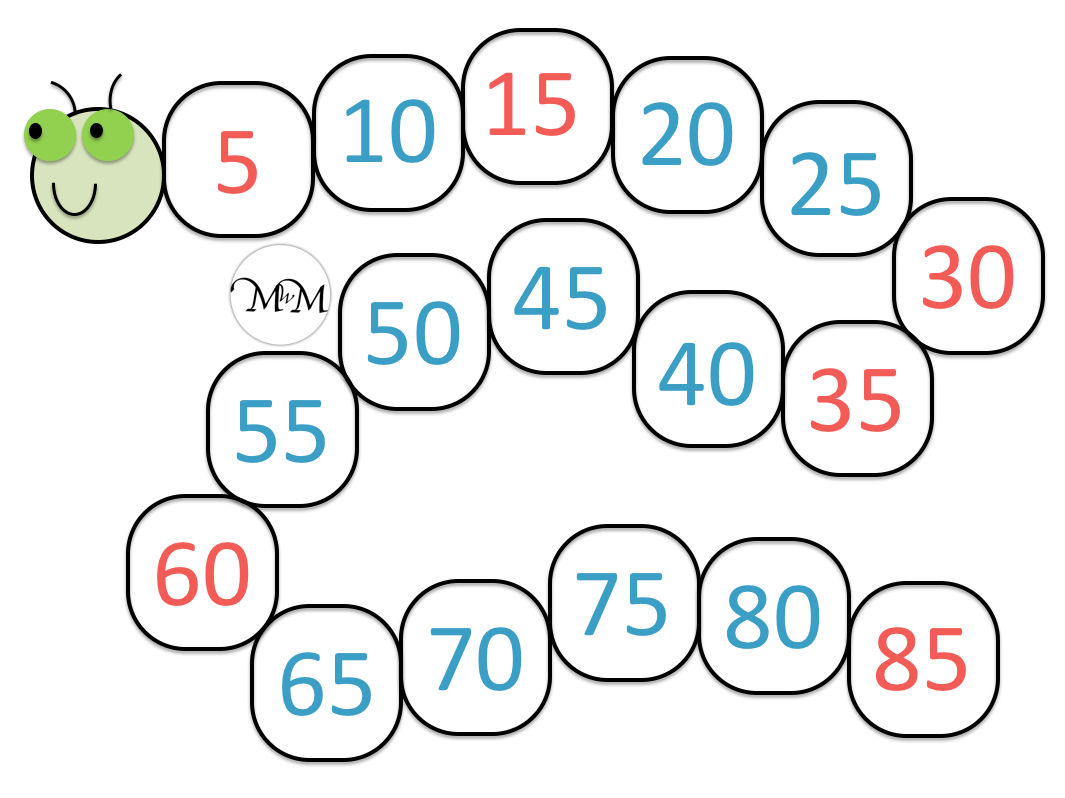## Skip Counting Backwards in Fives

To further practise skip counting by five, we can skip count backwards in fives.

We will start at 55 and skip count backwards by 5.

To skip count backwards in fives, subtract five each time to get to the next number.Skip counting backwards can help to reinforce the forwards pattern.

Remember that we have numbers ending in 5 and then 0. There is a number ending in 5 and 0 for each ten.

Subtracting 5 from and number ending in 0 in the units column results in a number ending in 5 that has a tens digit that is one less than the previous number.We have: 55 and 50, 45 and 40, 35 and 30, 25 and 20.

We will now look at an example of skip counting backwards in fives from 75.

Remember to count the numbers in pairs of number ending in 5 and 0 to help learn the pattern.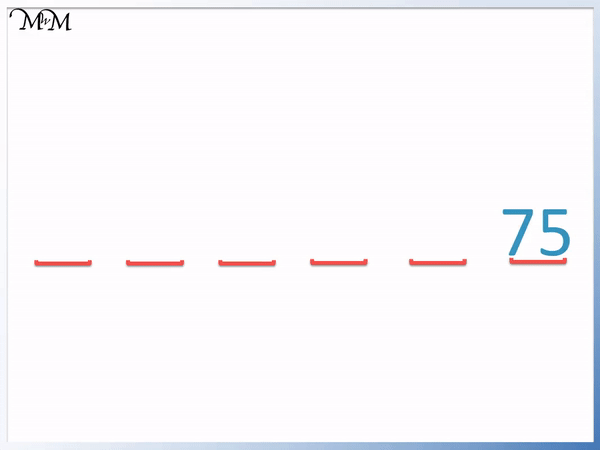We have 75 and 70, 65 and 60, 55 and 50.

Here is another skip counting caterpillar worksheet to practise skip counting backwards in fives.Here is the worksheet being completed, subtracting five each time to get to the next number.Now try our lesson on Skip Counting by 10 where we learn how to skip count in tens from any number.error: Content is protected !!